Question

# PD & PID controller design Consider a unity feedback system with open loop transfer function, G(s)...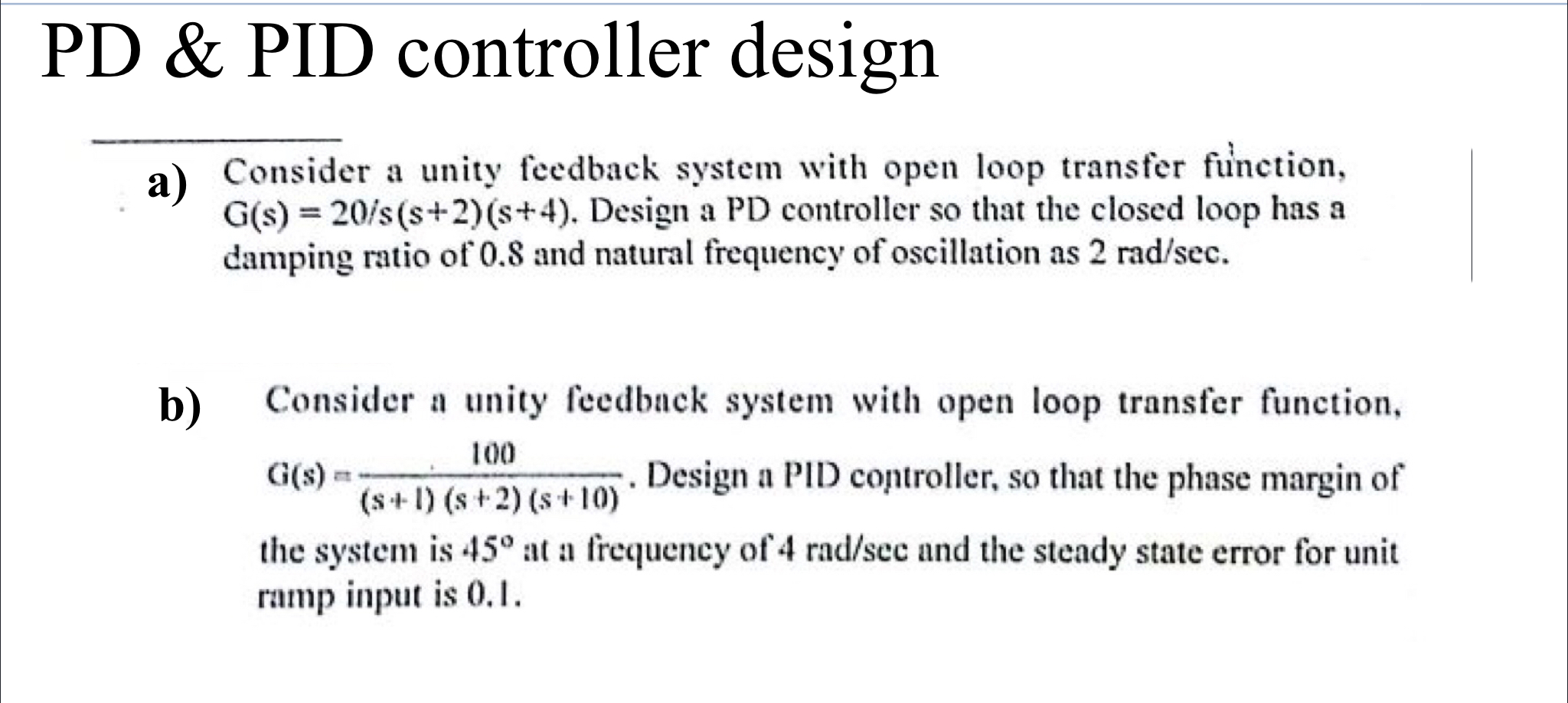PD & PID controller design Consider a unity feedback system with open loop transfer function, G(s) = 20/s(s+2)(8+4). Design a PD controller so that the closed loop has a damping ratio of 0.8 and natural frequency of oscillation as 2 rad/sec. b) 100 Consider a unity feedback system with open loop transfer function, aus. Design a PID controller, so that the phase margin of (S-1) (s + 2) (s+10) the system is 45° at a frequency of 4 rad/scc and the steady state error for unit ramp input is 0.1. G(s)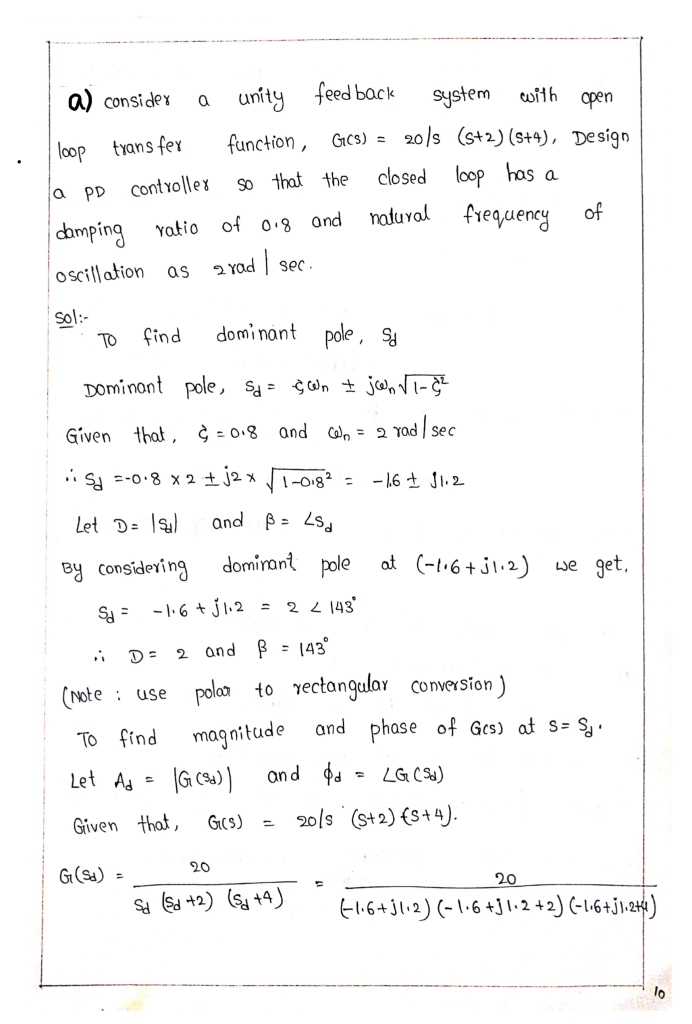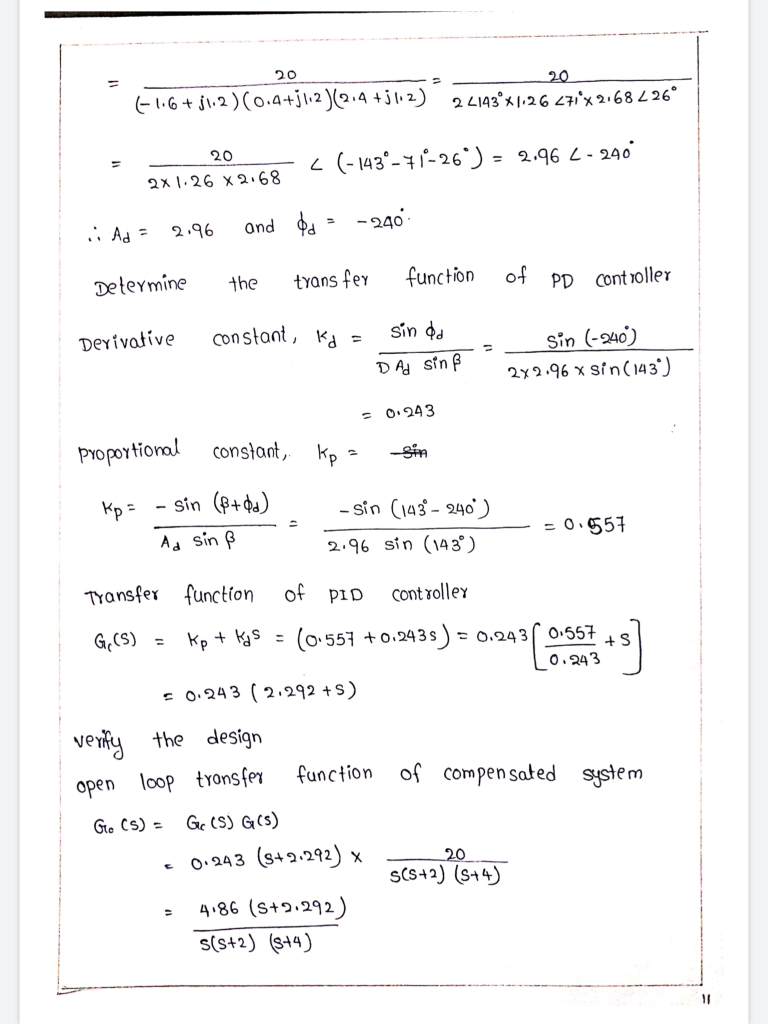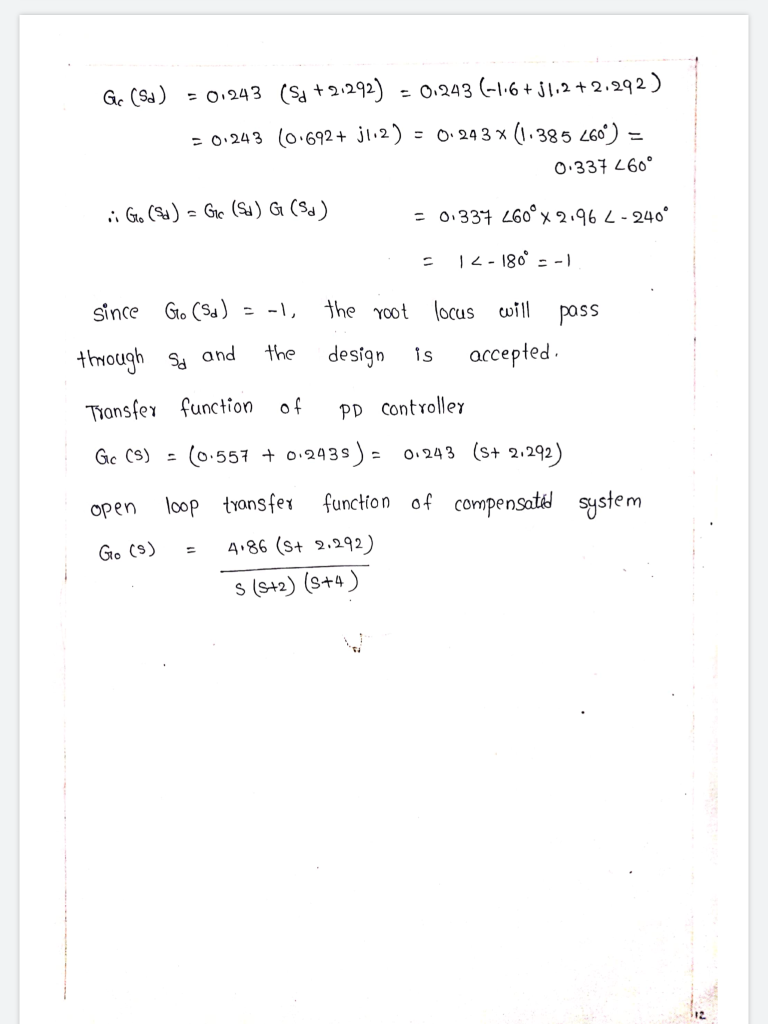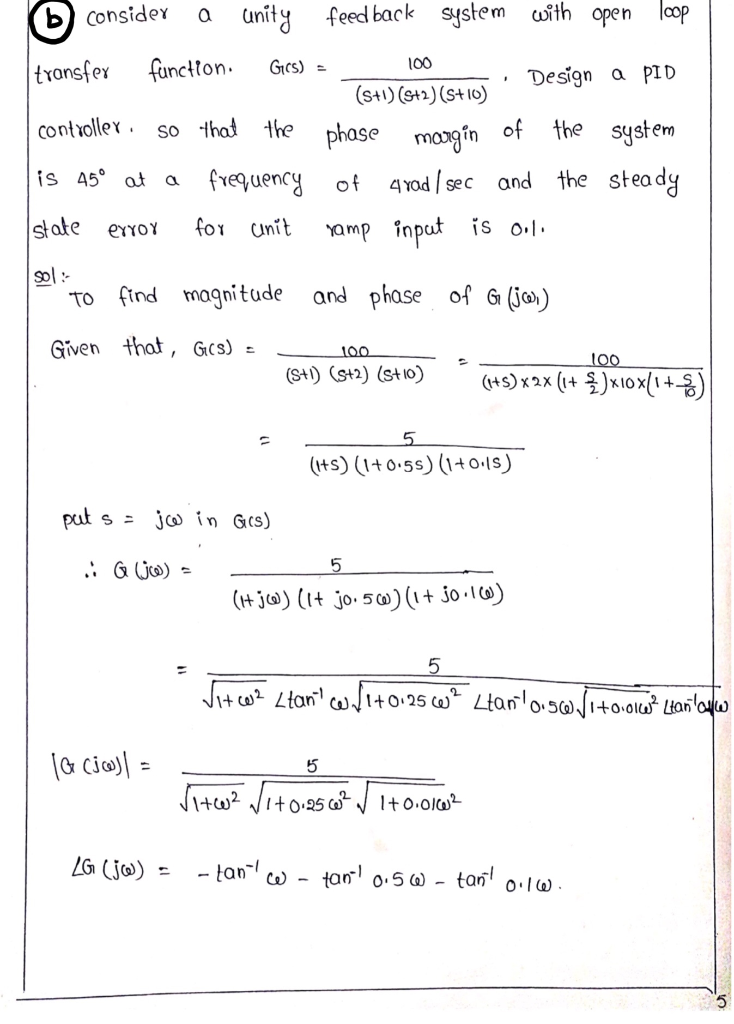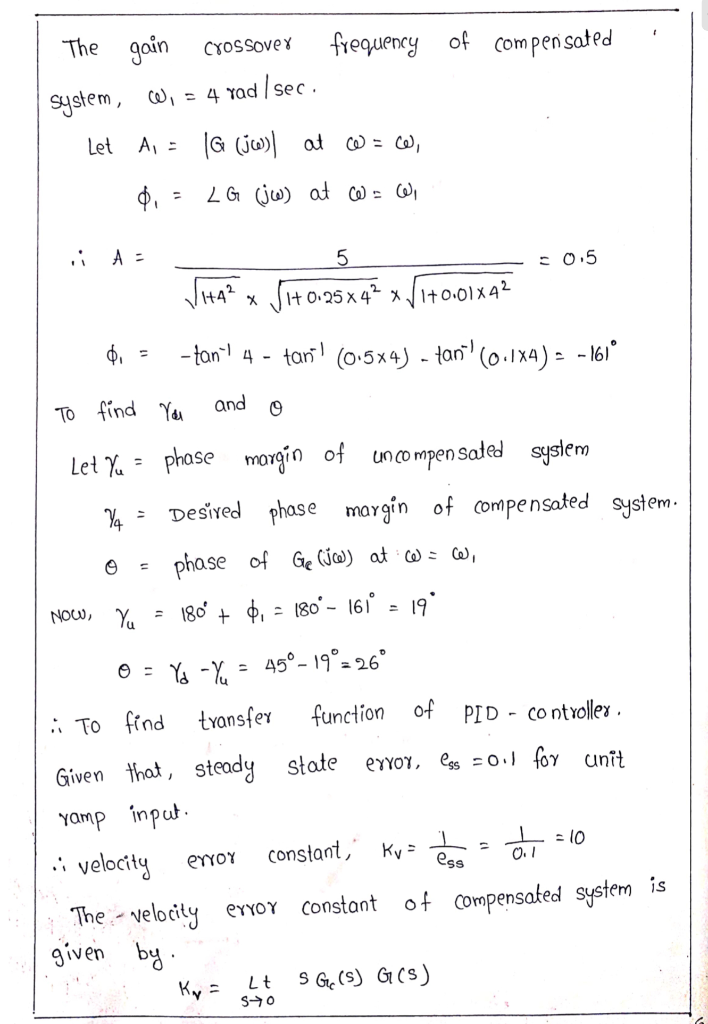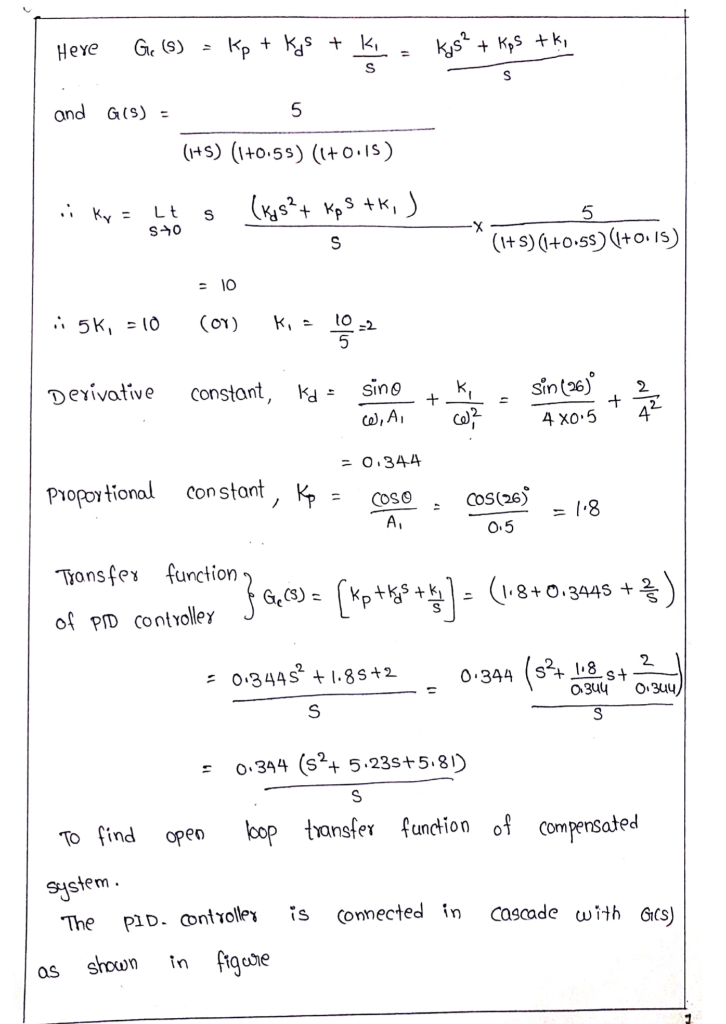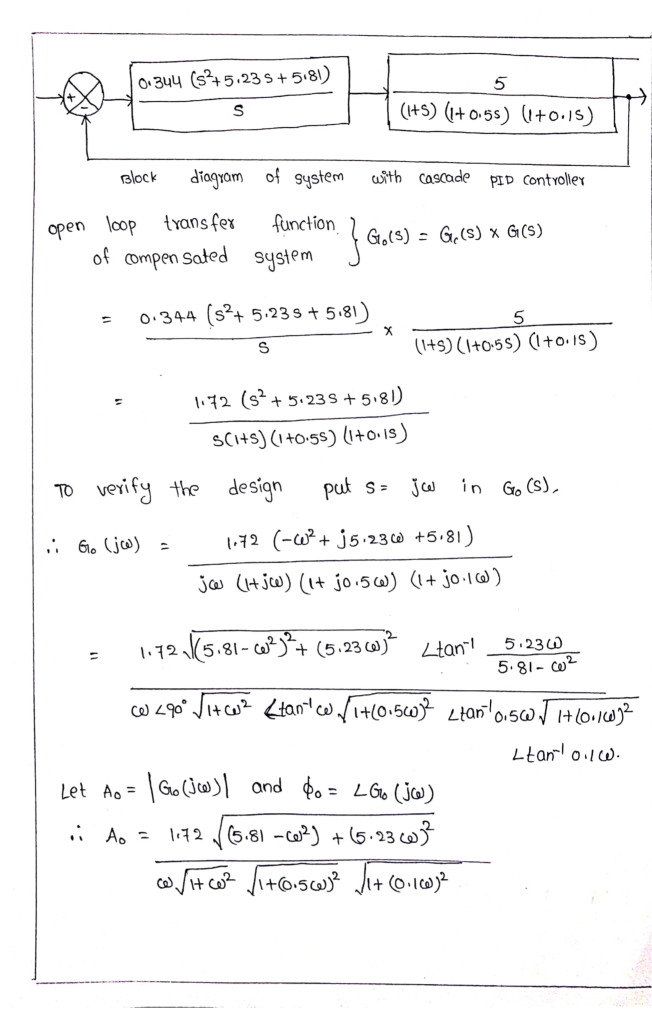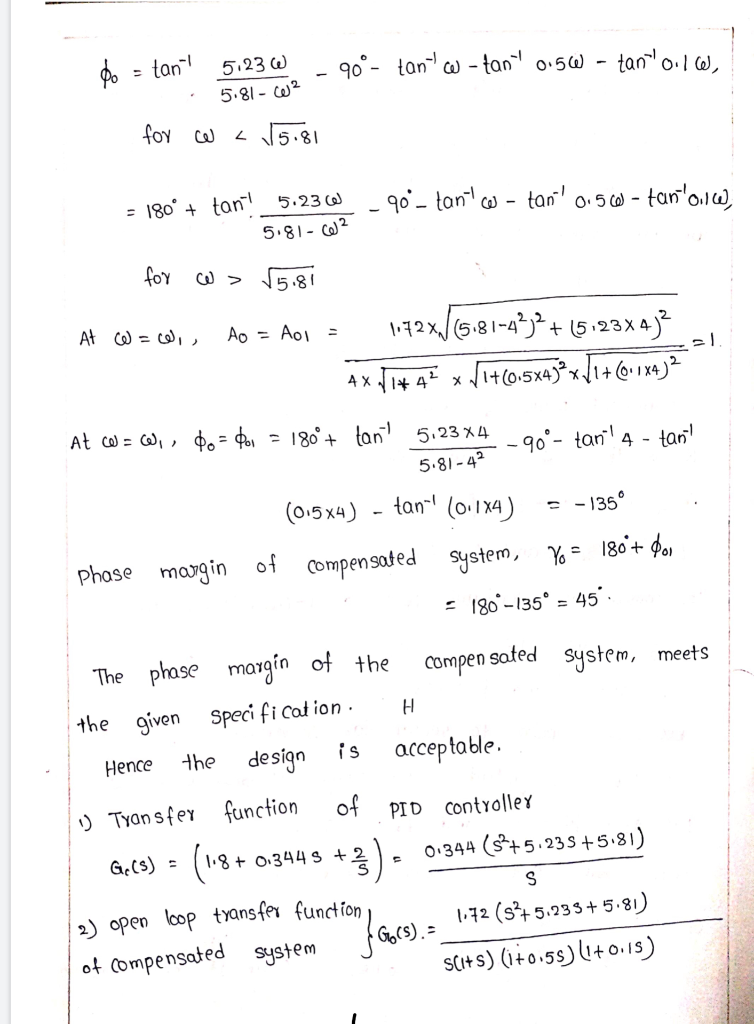#### Earn Coins

Coins can be redeemed for fabulous gifts.

Similar Homework Help Questions
• ### Q3. Consider a single loop unity feedback control system of the open loop transfer function (a) Find the range of values of the gain K and the parameter p so that: (i) The overshoot is less than 10%....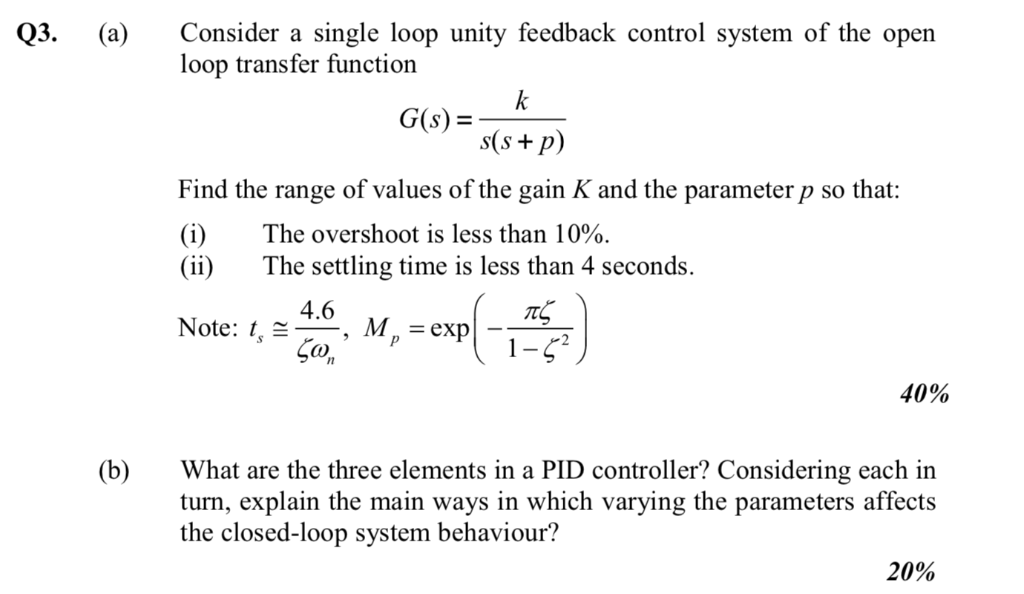Q3. Consider a single loop unity feedback control system of the open loop transfer function (a) Find the range of values of the gain K and the parameter p so that: (i) The overshoot is less than 10%. (ii)The settling time is less than 4 seconds Note: , 4.6 M. = exp CO 40% (b)What are the three elements in a PID controller? Considering each in turn, explain the main ways in which varying the parameters affects the closed-loop system...

• ### K and consider a PI s+4 A unity feedback system has an open loop transfer function G(s)  S+a controller Ge(s) S Sele...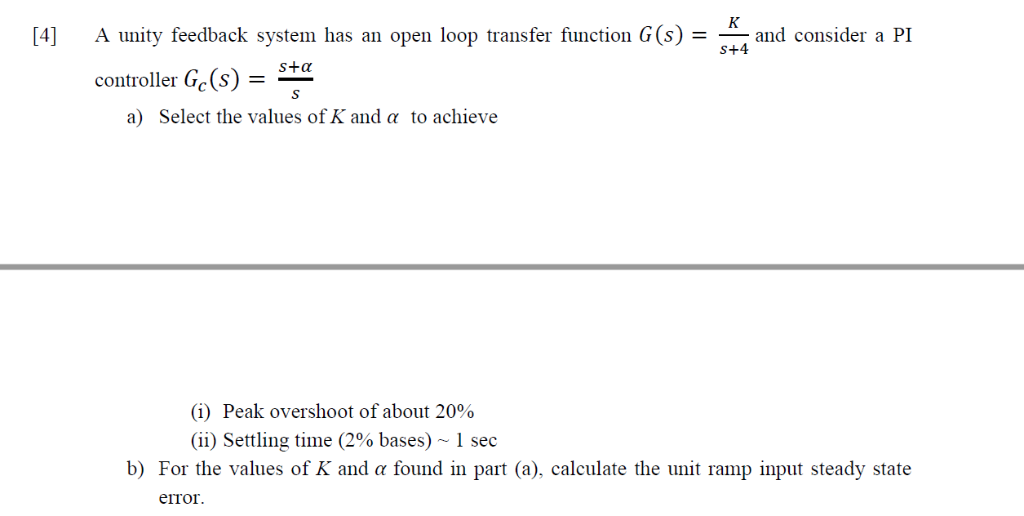K and consider a PI s+4 A unity feedback system has an open loop transfer function G(s)  S+a controller Ge(s) S Select the values of K and a to achieve a) (i) Peak overshoot of about 20% (ii) Settling time (2% bases) ~ 1 sec b) For the values of K and a found in part (a), calculate the unit ramp input steady state error K and consider a PI s+4 A unity feedback system has an open loop...

• ### urgent! II Lead-Lag Controller Design A plant has the open-loop transfer function with unity feedback: 20(s +1) G, (s) s(10s +D(0.1258 +D(0.05s +1)(0.02s +1) Design a phase lag-lead compensator th...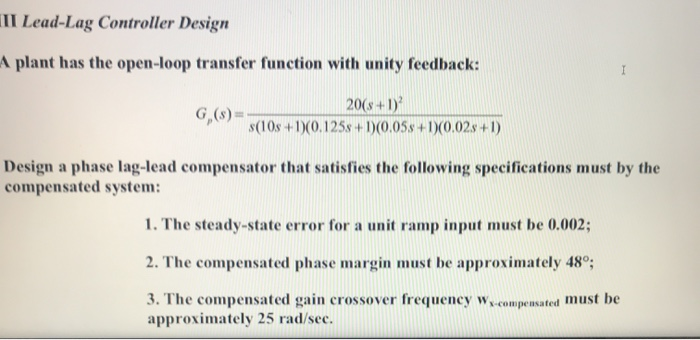urgent! II Lead-Lag Controller Design A plant has the open-loop transfer function with unity feedback: 20(s +1) G, (s) s(10s +D(0.1258 +D(0.05s +1)(0.02s +1) Design a phase lag-lead compensator that satisfies the following specifications must by the compensated system 1. The steady-state error for a unit ramp input must be 0.002; 2. The compensated phase margin must be approximately 48; must be approximately 25 rad/sec. II Lead-Lag Controller Design A plant has the open-loop transfer function with unity feedback: 20(s...

• ###  Sketch the root locus for the unity feedback system whose open loop transfer function is K G(s) Draw the root lo...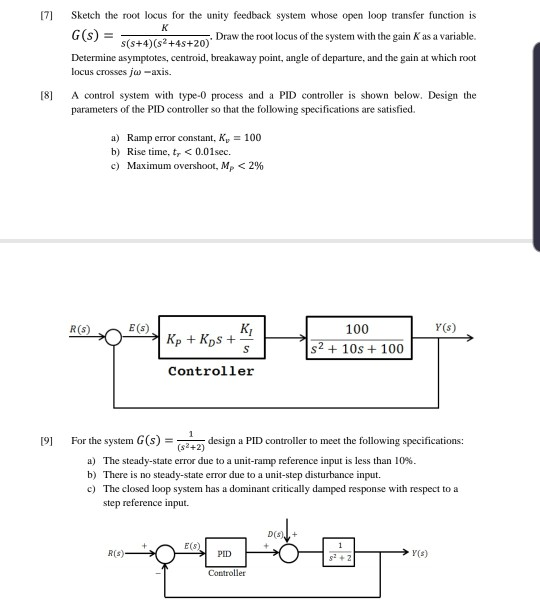Sketch the root locus for the unity feedback system whose open loop transfer function is K G(s) Draw the root locus of the system with the gain Kas a variable. s(s+4) (s2+4s+20) Determine asymptotes, centroid, breakaway point, angle of departure, and the gain at which root locus crosses ja-axis. A control system with type-0 process and a PID controller is shown below. Design the [8 parameters of the PID controller so that the following specifications are satisfied. =100 a)...

• ### 17. Consider unity feedback system with uncompensated forward transfer function a given by: K G(s) s+3)(s 6) The system...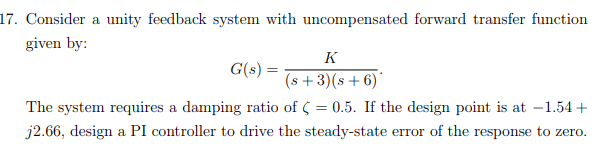17. Consider unity feedback system with uncompensated forward transfer function a given by: K G(s) s+3)(s 6) The system requires a damping ratio of 0.5. If the design point is at -1.54 j2.66, design a PI controller to drive the steady-state error of the response to zero 17. Consider unity feedback system with uncompensated forward transfer function a given by: K G(s) s+3)(s 6) The system requires a damping ratio of 0.5. If the design point is at -1.54 j2.66,...

• ### Problem 4. The open-loop transfer function of a unity feedback system is 20 G(s) S+1.5) (s +3.5) ...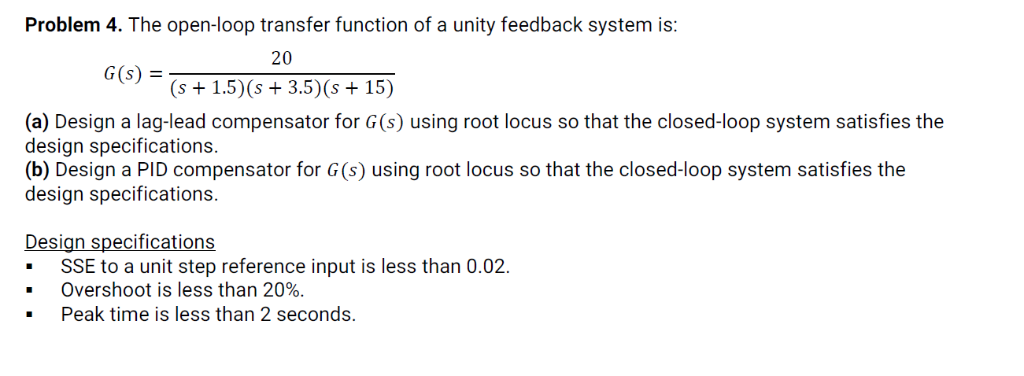Problem 4. The open-loop transfer function of a unity feedback system is 20 G(s) S+1.5) (s +3.5) (s +15) (a) Design a lag-lead compensator for G(s) using root locus so that the closed-loop system satisfies the design specifications. (b) Design a PID compensator for G(s) using root locus so that the closed-loop system satisfies the design specifications. Design specifications -SSE to a unit step reference input is less than 0.02. Overshoot is less than 20%. Peak time is less than...

• ### 4.35 Consider the feedback control system with the plant transfer function G(s) = (5+0.1)(5+0.5) (a) Design...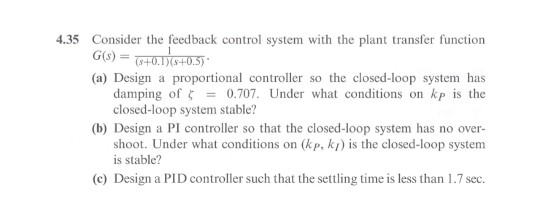4.35 Consider the feedback control system with the plant transfer function G(s) = (5+0.1)(5+0.5) (a) Design a proportional controller so the closed-loop system has damping of 5 = 0.707. Under what conditions on kp is the closed-loop system stable? (b) Design a PI controller so that the closed-loop system has no over- shoot. Under what conditions on (kp, kt) is the closed-loop system is stable? (©) Design a PID controller such that the settling time is less than 1.7 sec.

• ### please solve If a system has the open-loop transfer function G(s) s(s+25n) with unity feedback, then the closed-loop tr...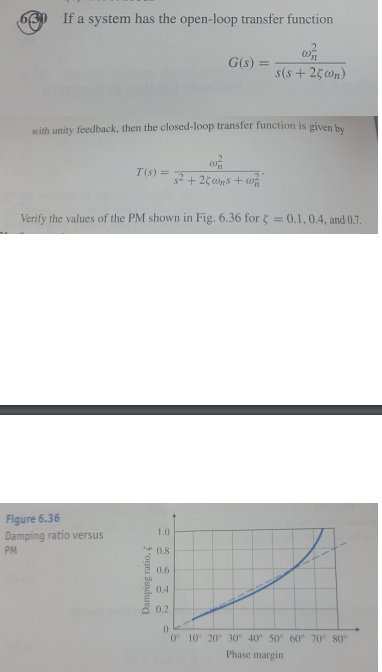please solve If a system has the open-loop transfer function G(s) s(s+25n) with unity feedback, then the closed-loop transfer function is given b T(s) s2+20ns+wf Verify the values of the PM shown in Fig. 6.36 for = 0.1,0.4, and 0.7. Figure 6.36 Damping ratio versus 1.0 0.8 PM 2 0.6 0,4 0.2 0 0° 10° 20° 30° 40° 50 60° 70° 80° Phase margin Damping ratio, If a system has the open-loop transfer function G(s) s(s+25n) with unity feedback, then...

• ### Problem 4. The open-loop transfer function of a unity feedback system is: 20 (s+1.5)(s 3.5) (s 15...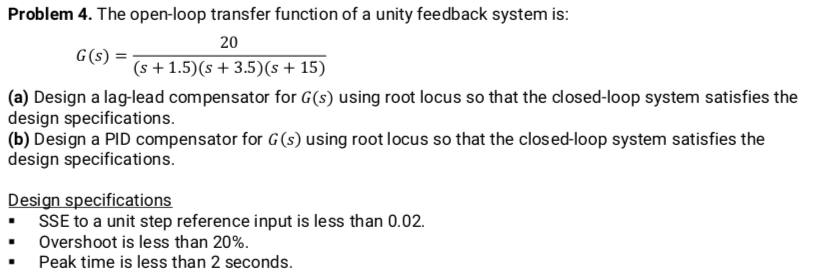Problem 4. The open-loop transfer function of a unity feedback system is: 20 (s+1.5)(s 3.5) (s 15) G(s) (a) Design a lag-lead compensator for G(s) using root locus so that the closed-loop system satisfies the design specifications (b) Design a PID compensator for G (s) using root locus so that the clos ed-loop system satisfies the design specifications. Design specifications .SSE to a unit step reference input is less than 0.02. Overshoot is less than 20% Peak time is less...

• ### 7. For a negative feedback control system with unit feedback gain, its open-loop 100 transfer function...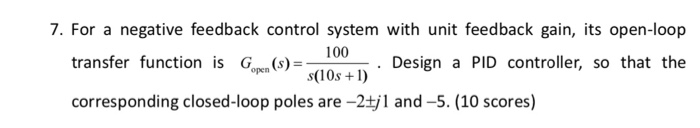7. For a negative feedback control system with unit feedback gain, its open-loop 100 transfer function is G (s) Design a PID controller, so that the open s(10s) corresponding closed-loop poles are -2+jl and -5. (10 scores) 7. For a negative feedback control system with unit feedback gain, its open-loop 100 transfer function is G (s) Design a PID controller, so that the open s(10s) corresponding closed-loop poles are -2+jl and -5. (10 scores)